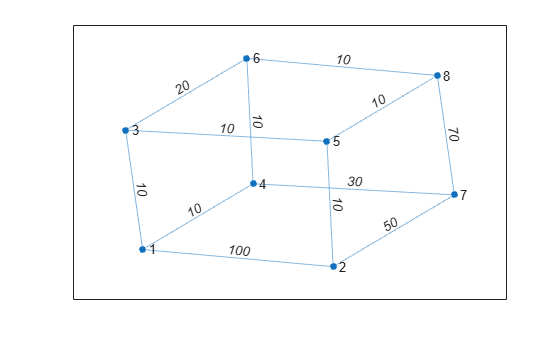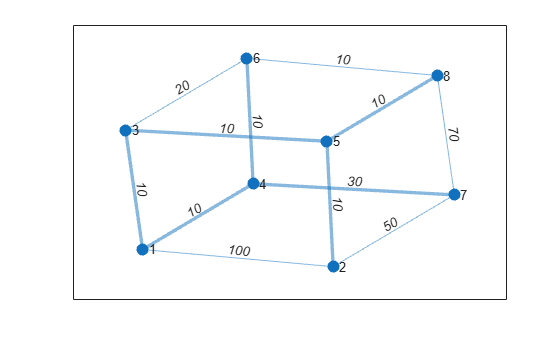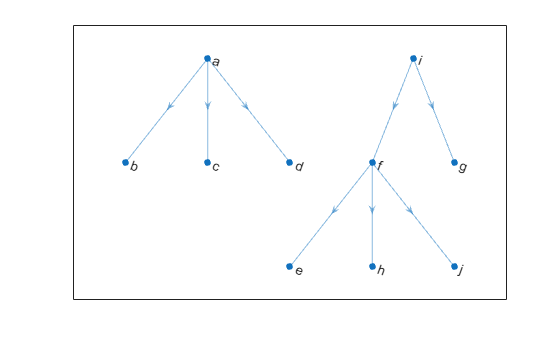# minspantree

Minimum spanning tree of graph

## Syntax

``T = minspantree(G)``
``T = minspantree(G,Name,Value)``
``````[T,pred] = minspantree(___)``````

## Description

example

````T = minspantree(G)` returns the minimum spanning tree, `T`, for graph `G`.```

example

````T = minspantree(G,Name,Value)` uses additional options specified by one or more Name-Value pair arguments. For example, `minspantree(G,'Method','sparse')` uses Kruskal’s algorithm for calculating the minimum spanning tree.```

example

``` ```[T,pred] = minspantree(___)``` also returns a vector of predecessor nodes, `pred`, using any of the input arguments in previous syntaxes.```

## Examples

collapse all

Create and plot a cube graph with weighted edges.

```s = [1 1 1 2 5 3 6 4 7 8 8 8]; t = [2 3 4 5 3 6 4 7 2 6 7 5]; weights = [100 10 10 10 10 20 10 30 50 10 70 10]; G = graph(s,t,weights); p = plot(G,'EdgeLabel',G.Edges.Weight);```Calculate and plot the minimum spanning tree of the graph on top of the graph. `T` contains the same nodes as `G`, but a subset of the edges.

```[T,pred] = minspantree(G); highlight(p,T)```Create and plot a graph that has multiple components.

```s = {'a' 'a' 'a' 'b' 'b' 'c' 'e' 'e' 'f' 'f' 'f' 'f' 'g' 'g'}; t = {'b' 'c' 'd' 'c' 'd' 'd' 'f' 'g' 'g' 'h' 'i' 'j' 'i' 'j'}; G = graph(s,t); p = plot(G,'Layout','layered');```Find the minimum spanning forest for the graph, starting at node `i`. Highlight the resulting forest in the plot. The graph node names are carried over into the minimum spanning tree graph.

```[T,pred] = minspantree(G,'Type','forest','Root',findnode(G,'i')); highlight(p,T)```Use the vector of predecessor nodes, `pred`, to create a directed version of the minimum spanning forest. All of the edges in this tree are directed away from the root nodes in each component (nodes `i` and `a`).

```rootedTree = digraph(pred(pred~=0),find(pred~=0),[],G.Nodes.Name); plot(rootedTree)```## Input Arguments

collapse all

Input graph, specified as a `graph` object. Use `graph` to create an undirected graph object.

Example: `G = graph(1,2)`

### Name-Value Pair Arguments

Specify optional comma-separated pairs of `Name,Value` arguments. `Name` is the argument name and `Value` is the corresponding value. `Name` must appear inside quotes. You can specify several name and value pair arguments in any order as `Name1,Value1,...,NameN,ValueN`.

Example: ```[T,pred] = minspantree(G,'Method','sparse')```

Minimum spanning tree algorithm, specified as the comma-separated pair consisting of `'Method'` and one of the options in the table.

OptionDescription
`'dense'` (default)Prim’s algorithm. This algorithm starts at the root node and adds edges to the tree while traversing the graph.
`'sparse'`Kruskal’s algorithm. This algorithm sorts all of the edges by weight, and then adds them to the tree if they do not create a cycle.

Root node, specified as the comma-separated pair consisting of `'Root'` and a node index or node name. The default root node is `1`.

• If `'Method'` is `'dense'` (default), then the root node is the starting node.

• If `'Method'` is `'sparse'`, then the root node is used only to compute `pred`, the vector of predecessor nodes.

You can specify the root node in any of these formats:

ValueExample
Scalar node index`1`
Character vector node name`'A'`
String scalar node name`"A"`

Type of minimum spanning tree, specified as the comma-separated pair consisting of `'Type'` and one of the options in the table.

OptionDescription
`'tree'`

Only a single tree is returned. The tree contains the root node.

`'forest'`

A forest of minimum spanning trees is returned. In other words, specify `'forest'` to calculate the minimum spanning tree of all connected components in the graph.

## Output Arguments

collapse all

Minimum spanning tree, returned as a `graph` object.

Predecessor nodes, returned as a vector of node indices. `pred(I)` is the node index of the predecessor of node `I`. By convention, ```pred(rootNode) = 0```. If `Type` is `'tree'`, then `pred(I) = NaN` for all nodes `I` that are not in the same component as the root node.

`pred` specifies a directed version of the minimum spanning tree, with all edges directed away from the root node.

collapse all

### Minimum Spanning Tree

For connected graphs, a spanning tree is a subgraph that connects every node in the graph, but contains no cycles. There can be many spanning trees for any given graph. By assigning a weight to each edge, the different spanning trees are assigned a number for the total weight of their edges. The minimum spanning tree is then the spanning tree whose edges have the least total weight.

For graphs with equal edge weights, all spanning trees are minimum spanning trees, since traversing `n` nodes requires `n-1` edges.